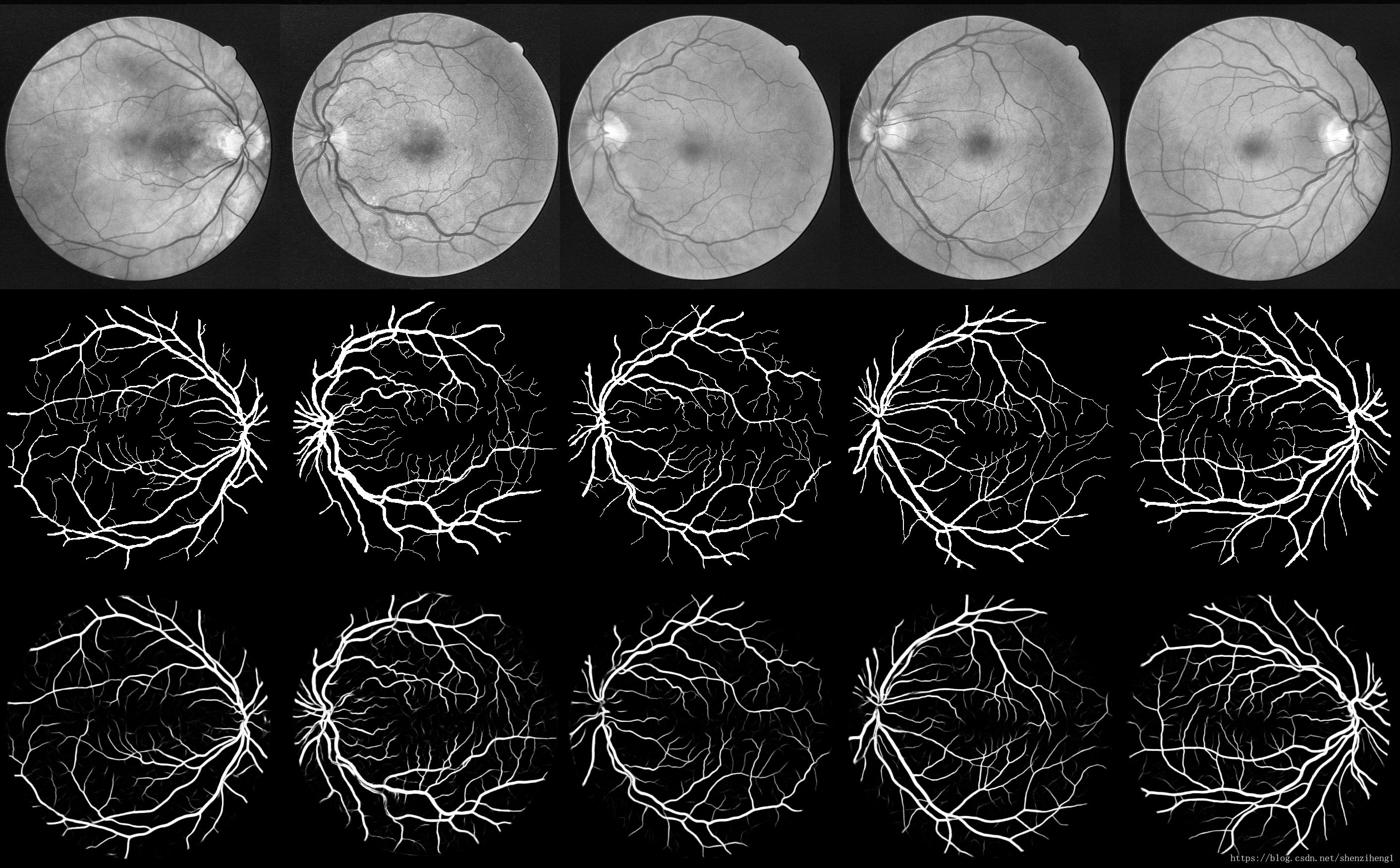# Unet项目解析(7): 模型编译-优化函数、损失函数、指标列表# 1. 模型编译

model.compile(optimizer='rmsprop',loss='categorical_crossentropy',metrics=['accuracy'])

• 损失函数loss：最小化的目标函数，为预定义的损失函数名，如categorical_crossentropy、mse，也可以为一个损失函数
• 指标列表metrics：对分类问题，一般设置为metrics=['accuracy']。指标可以是一个预定义指标的名字,也可以是一个用户定制的函数.指标函数应该返回单个张量,或一个完成metric_name - > metric_value映射的字典.

# 2. 优化器optimizer

# 调用model.compile()之前初始化一个优化器对象，然后传入该函数：
sgd = optimizers.SGD(lr=0.01, decay=1e-6, momentum=0.9, nesterov=True)
model.compile(loss='mean_squared_error', optimizer=sgd)

#在调用model.compile()时传递一个预定义优化器名
model.compile(loss='mean_squared_error', optimizer='sgd')

1. SGD：随机梯度下降法，支持动量参数，支持学习衰减率，支持Nesterov动量

keras.optimizers.SGD(lr=0.01, momentum=0.0, decay=0.0, nesterov=False)
# lr：大或等于0的浮点数，学习率
# momentum：大或等于0的浮点数，动量参数
# decay：大或等于0的浮点数，每次更新后的学习率衰减值
# nesterov：布尔值，确定是否使用Nesterov动量

2. RMSprop：该优化器通常是面对递归神经网络时的一个良好选择，除学习率可调整外，建议保持优化器的其他默认参数不变

keras.optimizers.RMSprop(lr=0.001, rho=0.9, epsilon=1e-06)
# lr：大或等于0的浮点数，学习率
# rho：大或等于0的浮点数
# epsilon：大或等于0的小浮点数，防止除0错误
keras.optimizers.Adagrad(lr=0.01, epsilon=1e-06)
# lr：大或等于0的浮点数，学习率
# epsilon：大或等于0的小浮点数，防止除0错误

keras.optimizers.Adadelta(lr=1.0, rho=0.95, epsilon=1e-06)
# lr：大或等于0的浮点数，学习率
# rho：大或等于0的浮点数
# epsilon：大或等于0的小浮点数，防止除0错误
keras.optimizers.Adam(lr=0.001, beta_1=0.9, beta_2=0.999, epsilon=1e-08)
# lr：大或等于0的浮点数，学习率
# beta_1/beta_2：浮点数， 0<beta<1，通常很接近1
# epsilon：大或等于0的小浮点数，防止除0错误

keras.optimizers.Nadam(lr=0.002, beta_1=0.9, beta_2=0.999, epsilon=1e-08, schedule_decay=0.004)
# lr：大或等于0的浮点数，学习率
# beta_1/beta_2：浮点数， 0<beta<1，通常很接近1
# epsilon：大或等于0的小浮点数，防止除0错误

# 3. 损失函数loss

• y_true：真实的数据标签，Theano/TensorFlow张量
• y_pred：预测值，与y_true相同shape的Theano/TensorFlow张量

## 3.1 封装好的目标函数

mean_squared_error或mse；mean_absolute_error或mae；mean_absolute_percentage_error或mape；mean_squared_logarithmic_error或msle；squared_hinge；hinge；categorical_hinge；binary_crossentropy（亦称作对数损失，logloss）；logcosh；categorical_crossentropy：亦称作多类的对数损失，注意使用该目标函数时，需要将标签转化为形如(nb_samples, nb_classes)的二值序列。sparse_categorical_crossentrop：同上，但接受稀疏标签。注意，使用该函数时仍然需要标签与输出值的维度相同，可能需要在标签数据上增加一个维度：np.expand_dims(y,-1)；kullback_leibler_divergence:从预测值概率分布Q到真值概率分布P的信息增益,用以度量两个分布的差异；poisson：即(predictions - targets * log(predictions))的均值；cosine_proximity：即预测值与真实标签的余弦距离平均值的相反数

## 3.2 自定义的目标函数

from keras import backend as K

def my_loss(y_true,y_pred):
return K.mean((y_pred-y_true),axis = -1)
model.compile(loss=my_loss,optimizer='SGD',metrics=['accuracy'])

# 4. 性能评估函数

# 可以通过字符串来使用域定义的性能评估函数
model.compile(loss='mean_squared_error',optimizer='sgd',metrics=['mae', 'acc'])

# 也可以自定义一个Theano/TensorFlow函数并使用之
from keras import metrics
model.compile(loss='mean_squared_error', optimizer='sgd', metrics=[metrics.mae, metrics.categorical_accuracy])

## 4.1 预定义评估函数

binary_accuracy: 对二分类问题,计算在所有预测值上的平均正确率
categorical_accuracy:对多分类问题,计算再所有预测值上的平均正确率
sparse_categorical_accuracy:与categorical_accuracy相同,在对稀疏的目标值预测时有用
top_k_categorical_accracy: 计算top-k正确率,当预测值的前k个值中存在目标类别即认为预测正确
sparse_top_k_categorical_accuracy：与top_k_categorical_accracy作用相同，但适用于稀疏情况

## 4.2 自定义评估函数

(y_true, y_pred) as arguments and return a single tensor value.
import keras.backend as K
def mean_pred(y_true, y_pred):
return K.mean(y_pred)
model.compile(optimizer='rmsprop', loss='binary_crossentropy', metrics=['accuracy', mean_pred])©️2019 CSDN 皮肤主题: 代码科技 设计师: Amelia_0503